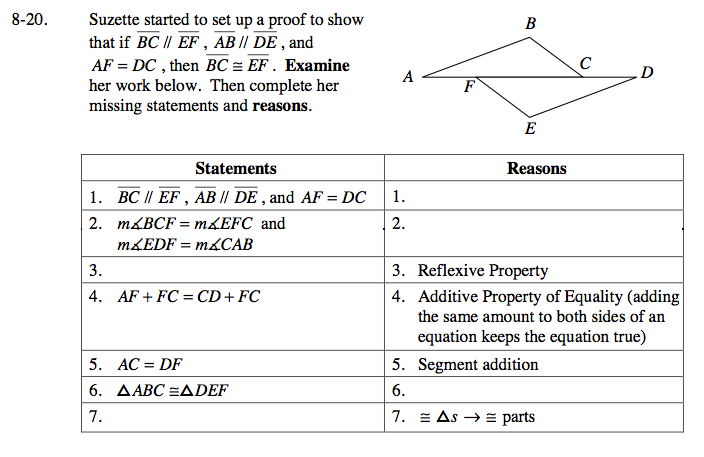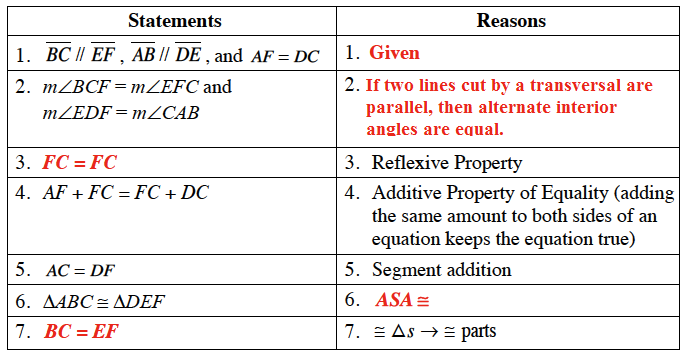Home > GC > Chapter 8 > Lesson 8.1.2 > Problem8-20

8-20.

Suzette started to set up a proof to show that if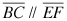,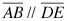and AF = DC , then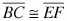. Examine her work below. Then complete her missing statements and reasons. Homework Help ✎

Statements

Reasons

1.,and AF = DC

1.

2. m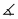BCF = mEFC and mEDF = mCAB

2.

3.

3. Reflexive Property

4. AF + FC = CD + FC

4. Additive Property of Equality (adding the same amount to both sides of an equation keeps the equation true)

5. AC = DF